Search by Topic

Resources tagged with Interactivities similar to Domino Join Up:

Filter by: Content type:
Age range:
Challenge level:

There are 197 results

Broad Topics > Information and Communications Technology > InteractivitiesMaking Trains

Age 5 to 7 Challenge Level:

Can you make a train the same length as Laura's but using three differently coloured rods? Is there only one way of doing it?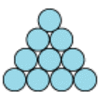Difference

Age 7 to 11 Challenge Level:

Place the numbers 1 to 10 in the circles so that each number is the difference between the two numbers just below it.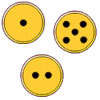Fair Exchange

Age 5 to 7 Challenge Level:

In your bank, you have three types of coins. The number of spots shows how much they are worth. Can you choose coins to exchange with the groups given to make the same total?Code Breaker

Age 7 to 11 Challenge Level:

This problem is based on a code using two different prime numbers less than 10. You'll need to multiply them together and shift the alphabet forwards by the result. Can you decipher the code?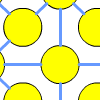One to Fifteen

Age 7 to 11 Challenge Level:

Can you put the numbers from 1 to 15 on the circles so that no consecutive numbers lie anywhere along a continuous straight line?A Square of Numbers

Age 7 to 11 Challenge Level:

Can you put the numbers 1 to 8 into the circles so that the four calculations are correct?Find the Difference

Age 5 to 7 Challenge Level:

Place the numbers 1 to 6 in the circles so that each number is the difference between the two numbers just below it.Junior Frogs

Age 5 to 11 Challenge Level:

Have a go at this well-known challenge. Can you swap the frogs and toads in as few slides and jumps as possible?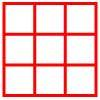Age 5 to 11 Challenge Level:

Place six toy ladybirds into the box so that there are two ladybirds in every column and every row.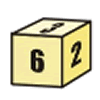First Connect Three for Two

Age 7 to 11 Challenge Level:

First Connect Three game for an adult and child. Use the dice numbers and either addition or subtraction to get three numbers in a straight line.One Million to Seven

Age 7 to 11 Challenge Level:

Start by putting one million (1 000 000) into the display of your calculator. Can you reduce this to 7 using just the 7 key and add, subtract, multiply, divide and equals as many times as you like?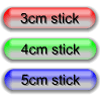Triangle Edges

Age 5 to 7 Challenge Level:

How many triangles can you make using sticks that are 3cm, 4cm and 5cm long?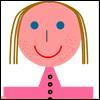The Brown Family

Age 5 to 7 Challenge Level:

Use the information about Sally and her brother to find out how many children there are in the Brown family.Counters

Age 7 to 11 Challenge Level:

Hover your mouse over the counters to see which ones will be removed. Click to remove them. The winner is the last one to remove a counter. How you can make sure you win?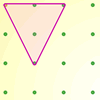Inside Triangles

Age 5 to 7 Challenge Level:

How many different triangles can you draw on the dotty grid which each have one dot in the middle?Arrangements

Age 7 to 11 Challenge Level:

Is it possible to place 2 counters on the 3 by 3 grid so that there is an even number of counters in every row and every column? How about if you have 3 counters or 4 counters or....?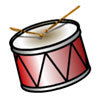We'll Bang the Drum

Age 5 to 7 Challenge Level:

How many different rhythms can you make by putting two drums on the wheel?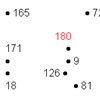A Dotty Problem

Age 7 to 11 Challenge Level:

Starting with the number 180, take away 9 again and again, joining up the dots as you go. Watch out - don't join all the dots!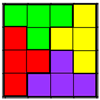Tetrafit

Age 7 to 11 Challenge Level:

A tetromino is made up of four squares joined edge to edge. Can this tetromino, together with 15 copies of itself, be used to cover an eight by eight chessboard?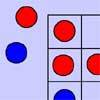Red Even

Age 7 to 11 Challenge Level:

You have 4 red and 5 blue counters. How many ways can they be placed on a 3 by 3 grid so that all the rows columns and diagonals have an even number of red counters?Multiples Grid

Age 7 to 11 Challenge Level:

What do the numbers shaded in blue on this hundred square have in common? What do you notice about the pink numbers? How about the shaded numbers in the other squares?Teddy Town

Age 5 to 14 Challenge Level:

There are nine teddies in Teddy Town - three red, three blue and three yellow. There are also nine houses, three of each colour. Can you put them on the map of Teddy Town according to the rules?Triangles All Around

Age 7 to 11 Challenge Level:

Can you find all the different triangles on these peg boards, and find their angles?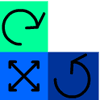Tessellate the Triominoes

Age 5 to 7 Challenge Level:

What happens when you try and fit the triomino pieces into these two grids?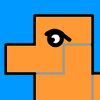Cover the Camel

Age 5 to 7 Challenge Level:

Can you cover the camel with these pieces?Seven Flipped

Age 7 to 11 Challenge Level:

Investigate the smallest number of moves it takes to turn these mats upside-down if you can only turn exactly three at a time.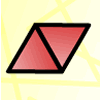Triangle Animals

Age 5 to 7 Challenge Level:

How many different ways can you find to join three equilateral triangles together? Can you convince us that you have found them all?Growing Garlic

Age 5 to 7 Challenge Level:

Ben and his mum are planting garlic. Use the interactivity to help you find out how many cloves of garlic they might have had.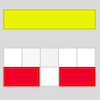Cuisenaire Counting

Age 5 to 7 Challenge Level:

Here are some rods that are different colours. How could I make a dark green rod using yellow and white rods?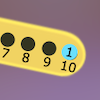Are You Well Balanced?

Age 5 to 7 Challenge Level:

Can you work out how to balance this equaliser? You can put more than one weight on a hook.Domino Numbers

Age 7 to 11 Challenge Level:

Can you see why 2 by 2 could be 5? Can you predict what 2 by 10 will be?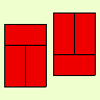Fault-free Rectangles

Age 7 to 11 Challenge Level:

Find out what a "fault-free" rectangle is and try to make some of your own.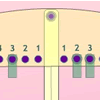Getting the Balance

Age 5 to 7 Challenge Level:

If you hang two weights on one side of this balance, in how many different ways can you hang three weights on the other side for it to be balanced?Four Triangles Puzzle

Age 5 to 11 Challenge Level:

Cut four triangles from a square as shown in the picture. How many different shapes can you make by fitting the four triangles back together?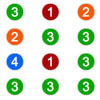Winning the Lottery

Age 7 to 11 Challenge Level:

Try out the lottery that is played in a far-away land. What is the chance of winning?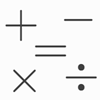Which Symbol?

Age 7 to 11 Challenge Level:

Choose a symbol to put into the number sentence.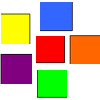Coloured Squares

Age 5 to 7 Challenge Level:

Use the clues to colour each square.More Transformations on a Pegboard

Age 7 to 11 Challenge Level:

Use the interactivity to find all the different right-angled triangles you can make by just moving one corner of the starting triangle.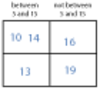More Carroll Diagrams

Age 7 to 11 Challenge Level:

How have the numbers been placed in this Carroll diagram? Which labels would you put on each row and column?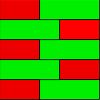Combining Cuisenaire

Age 7 to 11 Challenge Level:

Can you find all the different ways of lining up these Cuisenaire rods?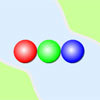Three Ball Line Up

Age 5 to 7 Challenge Level:

Use the interactivity to help get a feel for this problem and to find out all the possible ways the balls could land.Same Length Trains

Age 5 to 7 Challenge Level:

How many trains can you make which are the same length as Matt's, using rods that are identical?Age 7 to 11 Challenge Level:

Three beads are threaded on a circular wire and are coloured either red or blue. Can you find all four different combinations?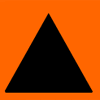Sorting Symmetries

Age 7 to 11 Challenge Level:

Find out how we can describe the "symmetries" of this triangle and investigate some combinations of rotating and flipping it.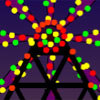Play a Merry Tune

Age 7 to 11 Challenge Level:

Explore the different tunes you can make with these five gourds. What are the similarities and differences between the two tunes you are given?Number Differences

Age 7 to 11 Challenge Level:

Place the numbers from 1 to 9 in the squares below so that the difference between joined squares is odd. How many different ways can you do this?Ring a Ring of Numbers

Age 5 to 7 Challenge Level:

Choose four of the numbers from 1 to 9 to put in the squares so that the differences between joined squares are odd.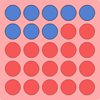All Change

Age 5 to 7 Challenge Level:

There are three versions of this challenge. The idea is to change the colour of all the spots on the grid. Can you do it in fewer throws of the dice?Nine-pin Triangles

Age 7 to 11 Challenge Level:

How many different triangles can you make on a circular pegboard that has nine pegs?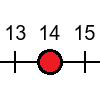Tug of War

Age 5 to 7 Challenge Level:

Can you use the numbers on the dice to reach your end of the number line before your partner beats you?